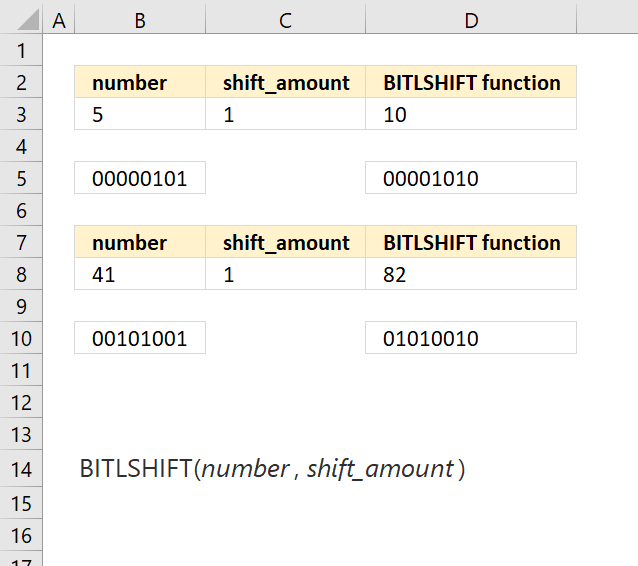Author: Oscar Cronquist Article last updated on May 11, 2022The BITLSHIFT function calculates a number whose binary representation is shifted left by a specified number of bits.

Formula in cell D3:

=BITLSHIFT(B3, C3)

Number 5 is 101 binary. Adding one zero from the right you get 1010 binary which is number 10.

### Excel Function Syntax

BITLSHIFT(numbershift_amount)

### Arguments

 number Required. The number you want to shift. shift_amount Required. How many zeros you want to add from the right.